#### Chapter 16 Circles R.D. Sharma Solutions for Class 9th Exercise 16.5

Exercise 16.5

1. In Fig. 16.176, ΔABC is an equilateral triangle. Find m∠BEC.Solution2. In Fig. 16.177, ΔPQR is an isosceles triangle with PQ = PR and m ∠PQR = 35°. Find m ∠QSR and m ∠QTR.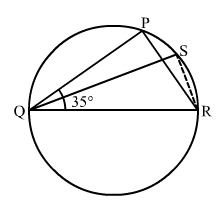Solution3. In Fig. 16.178, O is the centre of the circle. If ∠BOD = 160°, find the values of x and y.Solution4. In Fig. 16.179 ABCD is a cyclic quadrilateral. If ∠BCD = 100° and ∠ABD = 70°, find ∠ADB.Solution5. If ABCD is a cyclic quadrilateral in which AD || BC (Fig. 16.180). Prove that ∠B = ∠C.Solution6. In Fig. 16.181, O is the centre of the circle. Find ∠CBD.Solution7. In Fig. 16.182, AB and CD are diameters of a circle with centre O. If ∠OBD = 50°, find ∠AOC.Solution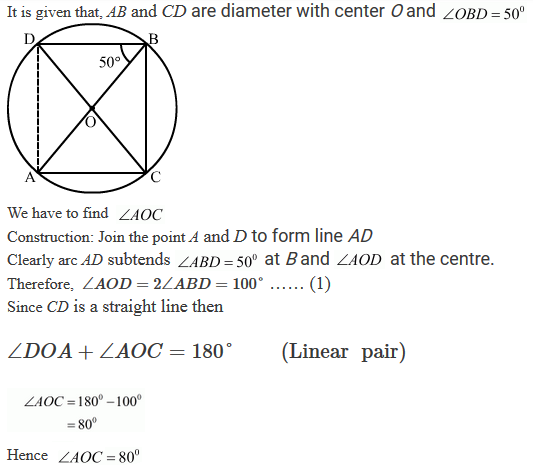8. On a semi-circle with AB as diameter, a point C is taken, so that m (∠CAB) = 30°. Find m (∠ACB) and m (∠ABC).

Solution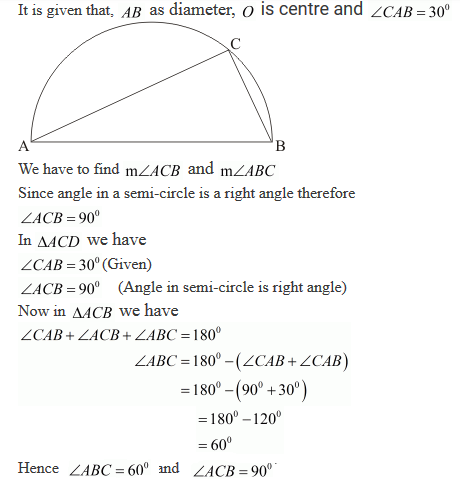9. In a cyclic quadrilateral ABCD if AB || CD and ∠B = 70°, find the remaining angles.

Solution10. In a cyclic quadrilateral ABCD, if m ∠A = 3 (m ∠C). Find m ∠A.

Solution11. In Fig. 16.183, O is the centre of the circle and ∠DAB = 50° . Calculate the values of x and y.Solution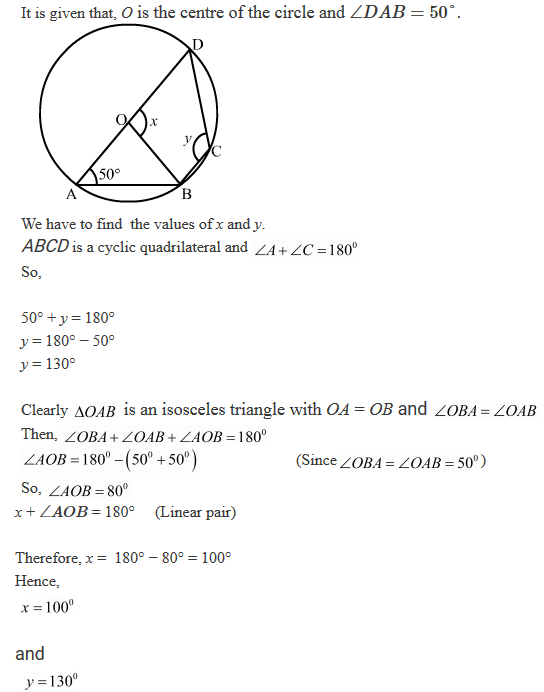12. In Fig. 16.184, if ∠BAC = 60° and ∠BCA = 20°, find ∠ADC.Solution13. In Fig. 16.185, if ABC is an equilateral triangle. Find ∠BDC and ∠BEC.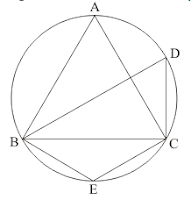Solution14. In Fig. 16.186, O is the centre of the circle. If ∠CEA = 30°, Find the values of x, y and z.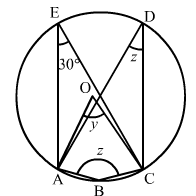Solution15. In Fig. 16.187, ∠BAD = 78°, ∠DCF = x° and ∠DEF = y°. Find the values of x and y.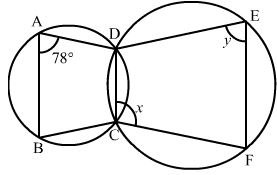Solution16. In a cyclic quadrilateral ABCD, if ∠A − ∠C = 60°, prove that the smaller of two is 60°

Solution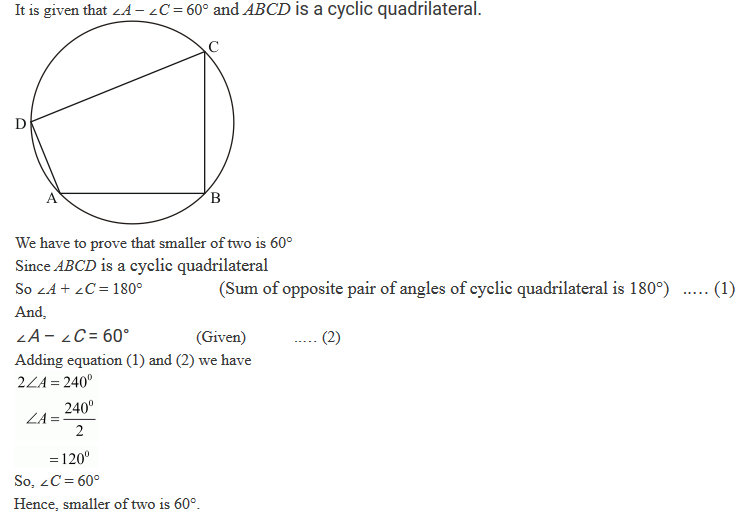17. In Fig. 16.188, ABCD is a cyclic quadrilateral. Find the value of x.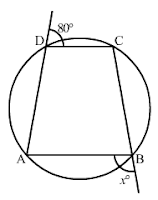Solution18. ABCD is a cyclic quadrilateral in which:
(i) BC || AD, ∠ADC = 110° and ∠BAC = 50°. Find ∠DAC.
(ii) ∠DBC = 80° and ∠BAC = 40°. Find ∠BCD.
(iii) ∠BCD = 100° and ∠ABD = 70° find ∠ADB.

Solution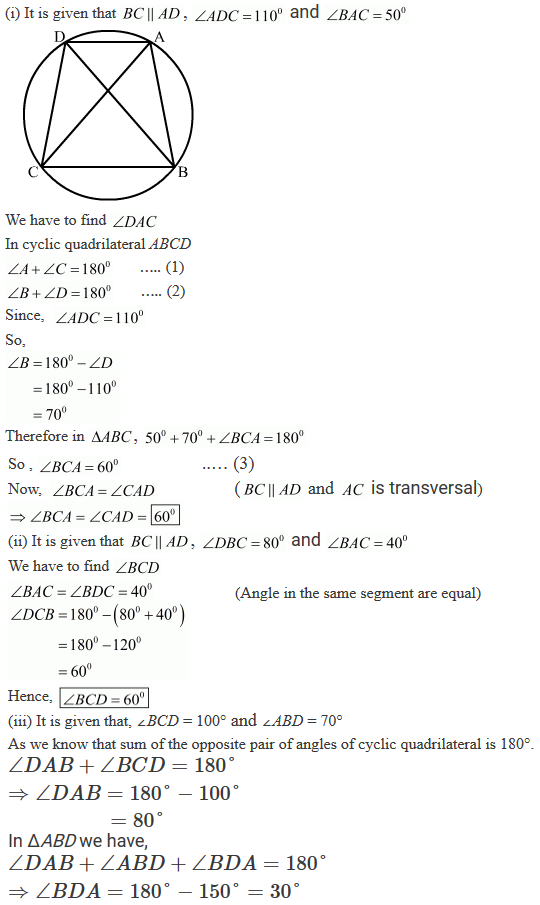19. Prove that the perpendicular bisectors of the sides of a cyclic quadrilateral are concurrent.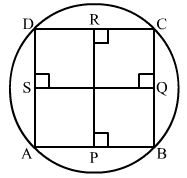To prove: Perpendicular bisector of side AB, BC, CD and DA are concurrent i.e, passes through the same point.
Proof:
We know that the perpendicular bisector of every chord of a circle always passes through the centre.
Therefore, Perpendicular bisectors of chord AB, BC, CD and DA pass through the centre which means they all passes through the same point.
Hence, the perpendicular bisector of AB, BC, CD and DA are concurrent.

20. Prove that the centre of the circle circumscribing the cyclic rectangle ABCD is the point of intersection of its diagonals.

Solution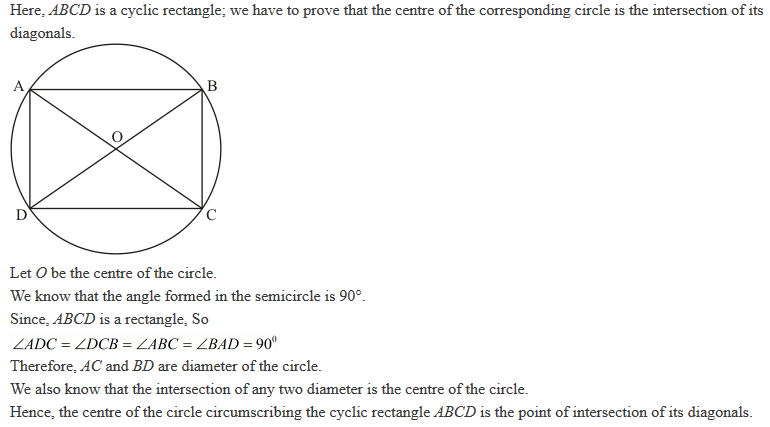21. Prove that the circles described on the four sides of a rhombus as diameters, pass through the point of intersection of its diagonals.

Solution22. If the two sides of a pair of opposite sides of a cyclic quadrilateral are equal, prove that its diagonals are equal.

Solution

∠ACB = ∠ADB  ---(2) (Angle in the same segment are equal)
In △ACD and △BDC,
CD = CD (common)
Hence, △ACD ≅ △BDC (SAS congruency criterion)
AC = BD (cpct)
Hence, Proved

23. ABCD is a cyclic quadrilateral in which BA and CD when produced meet in E and EA = ED. Prove that:
(ii) EB = EC.

Solution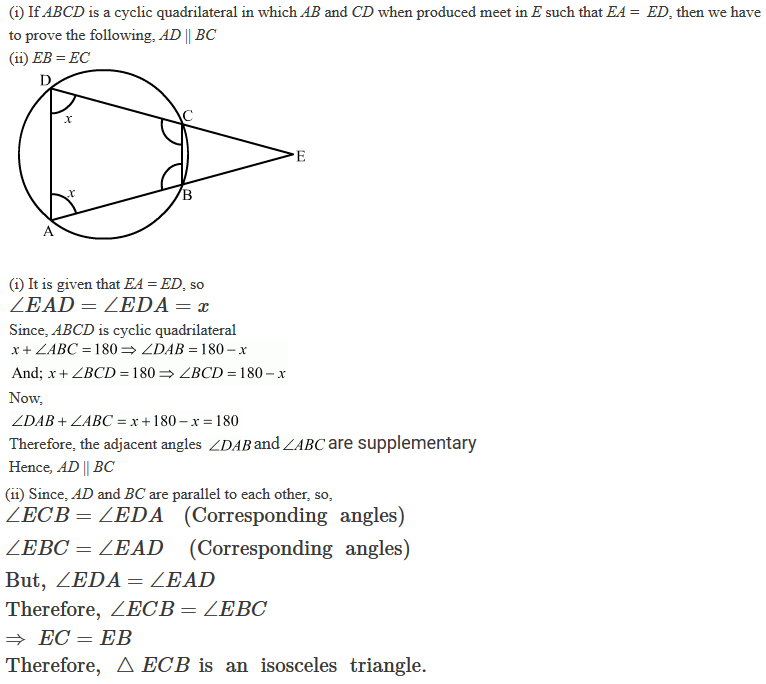24. Circles are described on the sides of a triangle as diameters. Prove that the circles on any two sides intersect each other on the third side (or third side produced).

Solution25. Prove that the angle in a segment greater than a semi-circle is less than a right angle.

Solution26. ABCD is a cyclic trapezium with AD || BC. If ∠B = 70°, determine other three angles of the trapezium.
Solution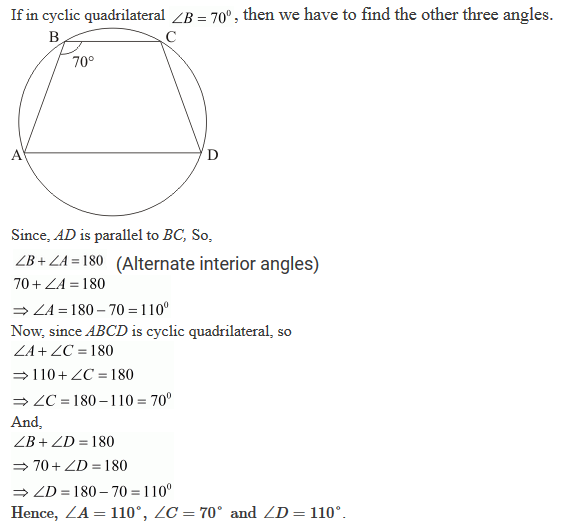27. Prove that the line segment joining the mid-point of the hypotenuse of a right triangle to its opposite vertex is half the hypotenuse.

Solution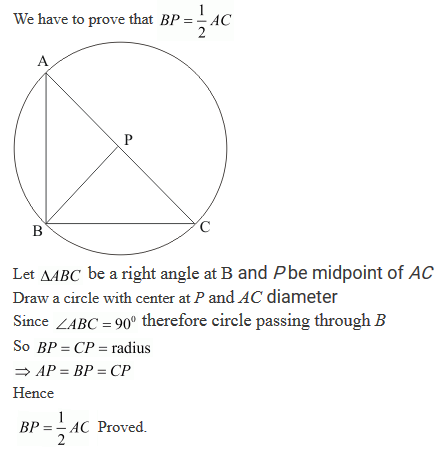28. In Fig. 16.189, ABCD is a cyclic quadrilateral in which AC and BD are its diagonals. If ∠DBC = 55° and ∠BAC = 45°, find ∠BCD.

Solution